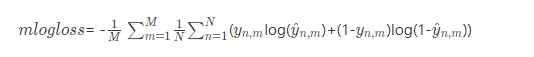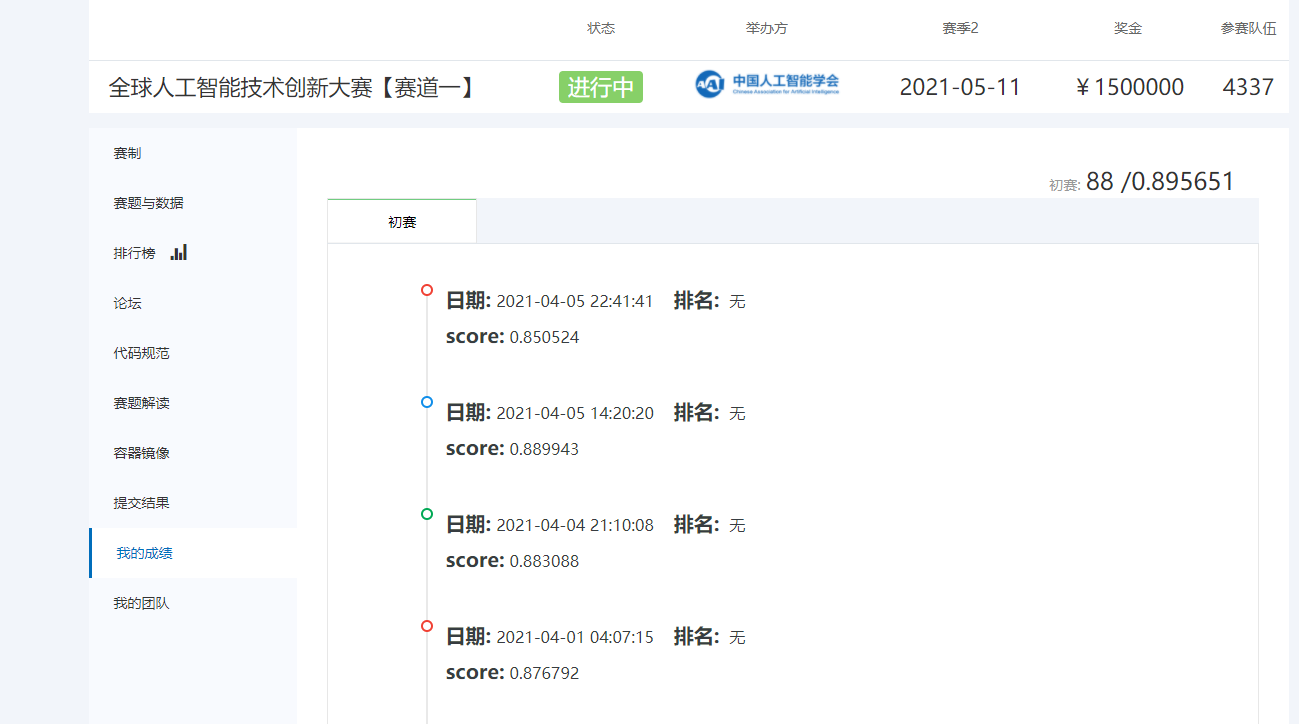• 医学影像报告
2021-01-25 20:55:36

### 目录

这是本人的目前的思考拙见，如果有问题，请在评论区指出，还在持续思考实时更新

# 详细赛题

【阿里天池赛题】2021年赛道一：医学影像报告异常检测

# 1 初赛赛题分析

（1）最多17个输入，但不固定，17类label，每个label0-1分类，但需要计算每个label的概率
样本数量为10份
训练集10000
测试集3000
（2）初赛是一个文本多标签多分类问题
sklearn包中的两个函数来计算预测结果和预测概率

• predict()：返回预测标签结果
• predict_proba()：预测属于某个标签的概率

# 2 赛题处理思路

（1）详细分析赛题
待完善。。。
（2）数据预处理

• 缺失值填充(pandas包)
• 异常值处理
• 是否有噪音，是否需要数据清洗
• 数据分布(Label类别分布、句子长度分布、统计特征的分布异常值、统计字段的缺失比例)+不均衡分布处理
（3）特征工程(特征值抽取)
• 脱敏数据特征提取
• 文本的向量处理（参考天池的新闻文本分类也是处理文本向量）
• 抽取的角度（需要头脑风暴）
• 特征处理(归一化、特征、标签编码)
（4）分类模型
• 分类算法：NB(Naive Bayes)，决策树、SVM、KNN、LR(Logistic Regression)等
• 神经网络：DL模型如CNN、LSTM、RNN等
当数据量不大，暂不优先考虑神经网络
（5）优化
• 算法优化
• 参数优化

# 3 准备工作

查找相关和类似赛题(关键词：文本分类、多分类、文本向量处理、文本向量神经网络、脱敏数据处理)

更多相关内容
• 全球人工智能技术创新大赛 赛道一: 医学影像报告异常检测ai
• 完全免费的CR、DR、CT、mr医学影像报告系统 灵活随意，
• 关于自动生成医学影像报告On the Automatic Generation of Medical Imaging Reports一个pytorch实现。 有关该文件的详细信息，请参见 。表现从模型only_training / only_training / 20180528-02：44：52 / 模式BLEU-...
• 天池大赛医学影像报告检测初赛26名代码分享（脱敏文本多标签分类） 介绍 数据：标签制作为one-hot形式，例如[3,4,6]就转为[0,0,0,1,1,0,1,…0]模型：采用nezha_large采用n-gram嵌入（具体见代码） 训练：将训练集，...
• 免费，好用，够用。比很多所谓大公司出的PACS系统里报告程序都要强大
• 基于pytorch实现了医学影像报告的自动生成 ./tensorboard.sh
• 影像科医生在工作时会观察医学影像（如CT、核磁共振影像），并对其作出描述，这些描述中包含了大量医学信息，对医疗AI具有重要意义。本任务需要参赛队伍根据医生对CT的影像描述文本数据，判断身体若干目标区域是否有...

天池-全球人工智能技术创新大赛

# 赛题背景

影像科医生在工作时会观察医学影像（如CT、核磁共振影像），并对其作出描述，这些描述中包含了大量医学信息，对医疗AI具有重要意义。本任务需要参赛队伍根据医生对CT的影像描述文本数据，判断身体若干目标区域是否有异常以及异常的类型。初赛阶段仅需判断各区域是否有异常，复赛阶段除了判断有异常的区域外，还需判断异常的类型。判断的结果按照指定评价指标进行评测和排名，得分最优者获胜。

# 赛题描述及数据说明

## sample数据

医生对若干CT的影像描述的明文数据，及描述中有异常区域与异常类型的label。样本数量为10份，以便使参赛队伍对比赛数据有直观的了解（Sample数据只是为了增进参赛选手对医疗影像描述的直观了解，实际训练与测试数据不一定与Sample数据具有相同特征或分布）。

每份样本占一行，使用分隔符“|,|”分割为3列，为不带表头的CSV数据格式。需要预测的人体区域有17个，复赛中需要判断的异常类型有12种。由于数据安全需要，不会告知具体区域与类型的名称，只会以ID表示，区域ID为0到16，类型ID为0到11。每个影像描述中可能有零个、一个或多个区域存在异常；若此描述有异常区域，则可能包含一个或多个异常类型。

## Training数据

脱敏后的影像描述与对应label。影像描述以字为单位脱敏，使用空格分割。初赛只进行各区域有无异常的判断，label只有异常区域ID。复赛除了判断各区域有无异常，还需要判断各区域异常的类型，因此label包含异常区域ID与异常类型ID。初赛Training集规模为10000例样本，复赛Training集规模为20000例样本。Training数据用于参赛选手的模型训练与预估。

• 初赛Training数据格式(不同列使用分隔符“|,|”分割）：
列名类型示例
report_IDint1
description脱敏后的影像描述，以字为单位使用空格分割101 47 12 66 74 90 0 411 234 79 175
label由多个异常区域ID组成，以空格分隔。若此描述中无异常区域，则为空3 4
• 复赛Training数据格式(不同列使用分隔符“|,|”分割）：
列名类型示例
report_IDint1
description脱敏后的影像描述，以字为单位使用空格分割101 47 12 66 74 90 0 411 234 79 175
labelstring，由两部分组成。第一部分为若干异常区域ID，用空格分割。第二部分为若干异常类型ID，用空格分割。两部分用逗号“,”分割。若定义中所有区域均无异常，则两部分均为空，此项为“,”。3 4,0 2

## Test数据

脱敏后的影像描述，脱敏方法和Training相同。Test数据用于参赛选手的模型评估和排名。初赛Test集分为AB榜，规模均为3000。复赛Test集规模为5000。

Test数据格式(不同列使用分隔符“|,|”分割）：

列名类型示例
report_IDint1
description脱敏后的影像描述，以字为单位使用空格分割101 47 12 66 74 90 0 411 234 79 175

# 提交说明

对于Test数据report_ID,description，选手应提交report_ID,prediction，其中prediction是预测结果。初赛中prediction是17维向量，值在0到1之间，表示各区域有异常的概率，使用空格分割。复赛中prediction是29维向量，值在0到1之间，前17个值表示17个区域有异常的概率，后12个值表示此描述包含各异常类型的概率。# 评估标准

在Test数据上将对选手提交结果使用ROC曲线的AUC（Area Under Curve）作为评估标准。下面以复赛分数为例，举例说明分数计算方法。为了便于说明，这里假设区域有3个而不是17个，异常类型有2个而不是12个。设真实值和选手提交的预测值分别如下：

真实值Ground Truth预测值Prediction
0 1 0 1 00 0.6 0.7 0.5 0
0 0 1 1 00 0.6 0.8 0.1 0.2

则第一部分分数为对以下数据计算AUC：# 比赛规则

1. 本项比赛全程不允许使用外部数据集。

2. 允许使用预训练模型，如网络模型与embedding等。

3. 复赛阶段可以使用初赛阶段的数据集。

展开全文• 中国医学影像行业报告：透视AI医学影像前景-爱分析-2019.6-50页.pdf
• 赛题：全球人工智能技术创新大赛赛道一: 医学影像报告异常检测 赛题背景 影像科医生在工作时会观察医学影像（如CT、核磁共振影像），并对其作出描述，这些描述中包含了大量医学信息，对医疗AI具有重要意义。本任务...

# 赛题：全球人工智能技术创新大赛赛道一: 医学影像报告异常检测

## 赛题背景

影像科医生在工作时会观察医学影像（如CT、核磁共振影像），并对其作出描述，这些描述中包含了大量医学信息，对医疗AI具有重要意义。本任务需要参赛队伍根据医生对CT的影像描述文本数据，判断身体若干目标区域是否有异常以及异常的类型。初赛阶段仅需判断各区域是否有异常，复赛阶段除了判断有异常的区域外，还需判断异常的类型。判断的结果按照指定评价指标进行评测和排名，得分最优者获胜。

## 赛题描述及数据说明

### sample数据

医生对若干CT的影像描述的明文数据，及描述中有异常区域与异常类型的label。样本数量为10份，以便使参赛队伍对比赛数据有直观的了解（Sample数据只是为了增进参赛选手对医疗影像描述的直观了解，实际训练与测试数据不一定与Sample数据具有相同特征或分布）。

每份样本占一行，使用分隔符“|,|”分割为3列，为不带表头的CSV数据格式。

列名类型示例
report_IDint1
descriptionstring，影像描述右下肺野见小结节样影与软组织肿块影
label由两部分组成。第一部分为若干异常区域ID，用空格分割。第二部分为若干异常类型ID，用空格分割。两部分用逗号“,”分割。若定义中所有区域均无异常，则两部分均为空，此项为“,”。4,1 2

需要预测的人体区域有17个，复赛中需要判断的异常类型有12种。由于数据安全需要，不会告知具体区域与类型的名称，只会以ID表示，区域ID为0到16，类型ID为0到11。每个影像描述中可能有零个、一个或多个区域存在异常；若此描述有异常区域，则可能包含一个或多个异常类型。

### Training数据

脱敏后的影像描述与对应label。影像描述以字为单位脱敏，使用空格分割。初赛只进行各区域有无异常的判断，label只有异常区域ID。复赛除了判断各区域有无异常，还需要判断各区域异常的类型，因此label包含异常区域ID与异常类型ID。初赛Training集规模为10000例样本，复赛Training集规模为20000例样本。Training数据用于参赛选手的模型训练与预估。

• 初赛Training数据格式(不同列使用分隔符“|,|”分割）：
列名类型示例
report_IDint1
description脱敏后的影像描述，以字为单位使用空格分割101 47 12 66 74 90 0 411 234 79 175
label由多个异常区域ID组成，以空格分隔。若此描述中无异常区域，则为空3 4
• 复赛Training数据格式(不同列使用分隔符“|,|”分割）：
列名类型示例
report_IDint1
description脱敏后的影像描述，以字为单位使用空格分割101 47 12 66 74 90 0 411 234 79 175
labelstring，由两部分组成。第一部分为若干异常区域ID，用空格分割。第二部分为若干异常类型ID，用空格分割。两部分用逗号“,”分割。若定义中所有区域均无异常，则两部分均为空，此项为“,”。3 4,0 2

## Test数据

脱敏后的影像描述，脱敏方法和Training相同。Test数据用于参赛选手的模型评估和排名。初赛Test集分为AB榜，规模均为3000。复赛Test集规模为5000。

Test数据格式(不同列使用分隔符“|,|”分割）：

列名类型示例
report_IDint1
description脱敏后的影像描述，以字为单位使用空格分割101 47 12 66 74 90 0 411 234 79 175

## 提交说明

对于Test数据report_ID,description，选手应提交report_ID,prediction，其中prediction是预测结果。初赛中prediction是17维向量，值在0到1之间，表示各区域有异常的概率，使用空格分割。复赛中prediction是29维向量，值在0到1之间，前17个值表示17个区域有异常的概率，后12个值表示此描述包含各异常类型的概率。

• 初赛提交数据格式(不同列使用分隔符“|,|”分割）：
列名类型示例
report_IDint1
Prediction17维向量0.68 0.82 0.92 0.59 0.71 0.23 0.45 0.36 0.46 0.64 0.92 0.66 0.3 0.5 0.94 0.7 0.38
• 复赛提交数据格式(不同列使用分隔符“|,|”分割）：
列名类型示例
report_IDint1
Prediction29维向量（中间不需要使用逗号分隔）0.68 0.82 0.92 0.59 0.71 0.23 0.45 0.36 0.46 0.64 0.92 0.66 0.3 0.5 0.94 0.7 0.38 0.05 0.97 0.71 0.5 0.64 0.0 0.54 0.5 0.49 0.41 0.06 0.07

## 评估标准

在Test数据上将对选手提交结果计算mlogloss作为评估标准，最终分数为1-mlogloss。
在初赛阶段，一个样本对应M(M=17)个预测值，N个样本共MN个预测值。对此MN个值的真实值与预测值计算mlogloss，计算方式如下：其中y_{n,m}yn,m​ 和\hat{y}_{n,m}y^​n,m​分别是第n个样本第m个标签的真实值和预测值。

初赛分数 S=1-mlogloss。为了让分数区间更合理，复赛阶段调整为S=1-2*mlogloss。

在复赛阶段，分数由两部分组成。第一部分与初赛相同，对预测值的前17维结合真实值计算S_1S1​得到 。第二部分为对所有实际存在异常区域的测试样本，对其预测值后12维结合真实异常类型进行计算，方法与第一部分相同，若N个测试样本中有K个实际有异常区域，则将对12K个值进行计算（实际无异常的样本不参与第二部分计算），得到S_2S2​。复赛最终分数S=0.6S_1+0.4S_2S=0.6S1​+0.4S2​。

# 开源方案

本方案根据线上84.7的开源分享(基于textCNN)-天池技术圈-天池技术讨论区，提供的Baseline做的修改，主要修改该了Mode、数据处理部分。baseline的特点：

本baselien基于textCNN构建

• 构建词汇表，总共858个词语，编号为0-857。
• 统一样本的长度，这里选择50个词语作为样本长度，多的截断，少的补齐(用858补齐)
• textCNN的第一层是对原始序列进行enmbeding，对每一个词都enmbed到固定维度，然后使用CNN来进行特征提取。
• 最后的输出采取BECWithlogitLoss()
• 线下验证指标采取auc和logloss两种方案

我主要做的修改：

• 统一样本的长度，这里选择64个词语作为样本长度，多的截断，少的补齐(用0补齐,用0补齐后大约有0.05的提高)
• textCNN的第一层是对原始序列进行enmbeding，对每一个词都enmbed到固定维度，然后使用CNN来进行特征提取，enmbeding改为64.

最终的初赛成绩是88，这个很奇怪，我记着当时已经下滑到100以外了，加上没有思路我就放弃了，错过一次进决赛的机会。## Net

详见：net.py

我总结一下我的Net的特点：

1、引入了3个TransformerEncoder编码器，我看到有人在kaggle的一个比赛top1方案中使用了，所以就拿过试试。

2、使用CNN的主力机制Coordinate Attention ，参考这篇： 注意力机制在CNN中使用总结_AI浩-CSDN博客

3、使用Mish激活函数。

4、构架多尺度的网络融合，和残差。

模型代码：

import torch
import torch.nn as nn
import torch.nn.functional as F
from collections import OrderedDict

channelNum = 64

class CA_Block(nn.Module):
def __init__(self, channel, h, w, reduction=16):
super(CA_Block, self).__init__()

self.h = h
self.w = w

self.avg_pool_x = nn.AdaptiveAvgPool2d((h, 1))
self.avg_pool_y = nn.AdaptiveAvgPool2d((1, w))

self.conv_1x1 = nn.Conv2d(in_channels=channel, out_channels=channel // reduction, kernel_size=1, stride=1,
bias=False)

self.relu = nn.ReLU()
self.bn = nn.BatchNorm2d(channel // reduction)

self.F_h = nn.Conv2d(in_channels=channel // reduction, out_channels=channel, kernel_size=1, stride=1,
bias=False)
self.F_w = nn.Conv2d(in_channels=channel // reduction, out_channels=channel, kernel_size=1, stride=1,
bias=False)

self.sigmoid_h = nn.Sigmoid()
self.sigmoid_w = nn.Sigmoid()

def forward(self, x):
x_h = self.avg_pool_x(x).permute(0, 1, 3, 2)
x_w = self.avg_pool_y(x)

x_cat_conv_relu = self.relu(self.conv_1x1(torch.cat((x_h, x_w), 3)))

x_cat_conv_split_h, x_cat_conv_split_w = x_cat_conv_relu.split([self.h, self.w], 3)

s_h = self.sigmoid_h(self.F_h(x_cat_conv_split_h.permute(0, 1, 3, 2)))
s_w = self.sigmoid_w(self.F_w(x_cat_conv_split_w))

out = x * s_h.expand_as(x) * s_w.expand_as(x)

return out

class Mish(torch.nn.Module):
def __init__(self):
super().__init__()

def forward(self, x):
x = x * (torch.tanh(torch.nn.functional.softplus(x)))
return x

class ConvBN(nn.Sequential):
def __init__(self, in_planes, out_planes, kernel_size, stride=1, groups=1):
if not isinstance(kernel_size, int):
padding = [(i - 1) // 2 for i in kernel_size]
else:
padding = (kernel_size - 1) // 2
super(ConvBN, self).__init__(OrderedDict([
('conv', nn.Conv2d(in_planes, out_planes, kernel_size, stride,
('bn', nn.BatchNorm2d(out_planes)),
# ('Mish', Mish())
('Mish', nn.LeakyReLU(negative_slope=0.3, inplace=False))
]))

class ResBlock(nn.Module):
"""
Sequential residual blocks each of which consists of \
two convolution layers.
Args:
ch (int): number of input and output channels.
nblocks (int): number of residual blocks.
shortcut (bool): if True, residual tensor addition is enabled.
"""

def __init__(self, ch, nblocks=1, shortcut=True):
super().__init__()
self.shortcut = shortcut
self.module_list = nn.ModuleList()
for i in range(nblocks):
resblock_one = nn.ModuleList()
resblock_one.append(ConvBN(ch, ch, 1))
resblock_one.append(Mish())
resblock_one.append(ConvBN(ch, ch, 3))
resblock_one.append(Mish())
self.module_list.append(resblock_one)

def forward(self, x):
for module in self.module_list:
h = x
for res in module:
h = res(h)
x = x + h if self.shortcut else h
return x

class Encoder_conv(nn.Module):
def __init__(self, in_planes=128, blocks=2, h=32, w=64):
super().__init__()
self.conv2 = ConvBN(in_planes, in_planes * 2, [1, 9])
self.conv3 = ConvBN(in_planes * 2, in_planes * 4, [9, 1])
self.conv4 = ConvBN(in_planes * 4, in_planes, 1)
self.resBlock = ResBlock(ch=in_planes, nblocks=blocks)
self.conv5 = ConvBN(in_planes, in_planes * 2, [1, 7])
self.conv6 = ConvBN(in_planes * 2, in_planes * 4, [7, 1])
self.conv7 = ConvBN(in_planes * 4, in_planes, 1)
self.eca = CA_Block(in_planes, h=h, w=w)
self.relu = Mish()

def forward(self, input):
x2 = self.conv2(input)
x3 = self.conv3(x2)
x4 = self.conv4(x3)
r1 = self.resBlock(x4)
x5 = self.conv5(r1)
x6 = self.conv6(x5)
x7 = self.conv7(x6)
x8 = self.relu(x7 + x4)
e = self.eca(x8)
return e

class TransformerEncoder(torch.nn.Module):
def __init__(self, embed_dim, num_heads, dropout, feedforward_dim):
super().__init__()
self.linear_1 = torch.nn.Linear(embed_dim, feedforward_dim)
self.linear_2 = torch.nn.Linear(feedforward_dim, embed_dim)
self.layernorm_1 = torch.nn.LayerNorm(embed_dim)
self.layernorm_2 = torch.nn.LayerNorm(embed_dim)

def forward(self, x_in):
attn_out, _ = self.attn(x_in, x_in, x_in)
x = self.layernorm_1(x_in + attn_out)
ff_out = self.linear_2(torch.nn.functional.relu(self.linear_1(x)))
x = self.layernorm_2(x + ff_out)
return x

class CNN_Text(nn.Module):

def __init__(self, embed_num, static=False):
super(CNN_Text, self).__init__()
embed_dim = 128
class_num = 17
Ci = 1
self.embed = nn.Embedding(embed_num, embed_dim)  # 词嵌入
self.tram = TransformerEncoder(embed_dim, 8, 0.5, 512)
self.encoder_2 = TransformerEncoder(embed_dim, 8, 0.5, 512)
self.encoder_3 = TransformerEncoder(embed_dim, 8, 0.5, 512)
self.encoder1 = nn.Sequential(OrderedDict([
("conv3_bn3", ConvBN(Ci, channelNum, 1)),
("encoder_conv1", Encoder_conv(channelNum, blocks=2, h=64, w=128)),
]))
self.encoder2 = nn.Sequential(OrderedDict([
("conv3_bn_3,3", ConvBN(Ci, channelNum * 2, 3)),
("conv3_bn1,1", ConvBN(channelNum * 2, channelNum // 2, 1)),
('se', CA_Block(channelNum // 2, h=64, w=128)),
]))
self.encoder3 = nn.Sequential(OrderedDict([
("conv3_bn3", ConvBN(Ci, channelNum * 2, [1, 3])),
("conv3_bn31", ConvBN(channelNum * 2, channelNum // 2, [3, 1])),
('se', CA_Block(channelNum // 2, h=64, w=128)),
]))
self.encoder_conv = Encoder_conv(channelNum * 2)
self.encoder_conv1 = nn.Sequential(OrderedDict([
("conv1x1_bn", ConvBN(channelNum * 2, 1, 1)),
]))
self.con1 = ConvBN(Ci, channelNum * 2, 1, stride=2)
self.relu = Mish()
self.pool = nn.AvgPool2d(2);
self.fc1 = nn.Linear(2048, class_num)
self.dp = nn.Dropout(0.5)
self.sg = nn.Sigmoid()
if static:

def forward(self, x):
x = self.embed(x)  # (N, W, D)-batch,单词数量，维度
x1=self.tram(x)
x2=self.encoder_2(x1)
x = self.encoder_3(x2)
x = x.unsqueeze(1)  # (N, Ci, W, D)
x = self.sg(x)
x0 = self.con1(x)
encode1 = self.encoder1(x)
encode2 = self.encoder2(x)
encode3 = self.encoder3(x)
x = torch.cat((encode1, encode2, encode3), dim=1)
x = self.relu(x)
x = self.pool(x)
x = self.encoder_conv(x)
x = self.relu(x + x0)
x = self.encoder_conv1(x)
x = x.contiguous().view(-1, 2048)
x = self.dp(x)
logit = self.fc1(x)  # (N, C)
return logit

if __name__ == "__main__":
net = CNN_Text(embed_num=1000)
x = torch.LongTensor([[1, 2, 4, 5, 2, 35, 43, 113, 111, 451, 455, 22, 45, 55],
[14, 3, 12, 9, 13, 4, 51, 45, 53, 17, 57, 954, 156, 23]])
logit = net(x)
print(net)


## 数据分析

详见eda.py

我在数据分析上做的主要工作有：

1、数据长度的分析，数据最长是104，最短是4，平均值是41.

2、找出高频词，并在加载数据时，将高频词去除。（此操作不但没有提升分数，反而下降了，不学科啊）

import pandas as pd
import numpy as np
from collections import Counter
#
#
train_df.columns=['report_ID','description','label']
test_df.columns=['report_ID','description']
train_df.drop(['report_ID'],axis=1,inplace=True)
test_df.drop(['report_ID'],axis=1,inplace=True)
print("train_df:{},test_df:{}".format(train_df.shape,test_df.shape))
#
new_des=[i.strip('|').strip() for i in train_df['description'].values]
new_label=[i.strip('|').strip() for i in train_df['label'].values]
train_df['description']=new_des
train_df['label']=new_label
new_des_test=[i.strip('|').strip() for i in test_df['description'].values]
test_df['description']=new_des_test
#
word_all=[]
len_list=[]
for i in range(len(new_des)):
tmp=[int(i) for i in new_des[i].split(' ')]
word_all+=tmp
len_list.append(len(tmp))
for i in range(len(new_des_test)):
tmp=[int(i) for i in new_des_test[i].split(' ')]
word_all+=tmp
len_list.append(len(tmp))
#
print(train_df['label'].unique())
a=Counter(word_all)
print(len(a))
a=dict(a)
a=sorted(a)#0-857
#print(a)
print(np.max(len_list),np.min(len_list),np.mean(len_list))

## 训练

这里面我对loss做了修改，本次loss如下：pytorch中最接近的的loss是torch.nn.BCEWithLogitsLoss()，但是在计算有差别，所以我尝试自己写loss。

def logloss(y_true, y_pred):
# Clip y_pred between eps and 1-eps
p = torch.clamp(y_pred, 1e-5, 1-1e-5)
loss = torch.sum(y_true * torch.log(p) + (1 - y_true) * torch.log(1 - p))
return loss / len(y_true)

class Muti_logloss(torch.nn.Module):
def __init__(self):
super(Muti_logloss, self).__init__()

def forward(self, y, y_p):
allloss = []
for i in range(y.shape):
loss = logloss(y[:, i], y_p[:, i])
allloss.append(loss)
allloss = torch.tensor(allloss, dtype=torch.float)
alllosssum = torch.sum(allloss)
lossre = alllosssum / (y.shape)
lossre = -Variable(lossre, requires_grad=True)
return lossre



写完loss后，接着训练，发现loss一直不收敛，找了几个大佬帮我核对loss没有问题。不知道哪里出问题了，先记录。最后还是用的 BCEWithLogitsLoss()。

完整的代码连接：

医学影像报告异常检测0.8956.zip-深度学习文档类资源-CSDN下载

展开全文pytorch 神经网络 数据挖掘 机器学习
• 2021年中国人工智能医学影像企业发展报告（102页）.pdf
• 医学影像设备学 诊断X线机简介 包括胃肠X线机 摄影X线机 其他专用X线机
• 2021年中国AI医学影像行业研究报告（47页）.pdf
• 未来的医学影像将是精准医学的重要组成部分。美国国家医学科学院（IOM）在2011年11月发出了一份报告，特别强调精准医学是建立在了解个体基因、环境、生活方式的基础上的新型的疾病治疗和预防的方法。我们利用生命...
• 数据挖掘在医学影像储存与传输系统结构化报告中的应用.pdf
• 医学影像专业述职报告 医学影像技术好就业.doc
• 2021年中国人工智能医学影像企业发展报告（104页）.pdf人工智能
• 医学影像设备学教学课件医学影像设备学概论.ppt...